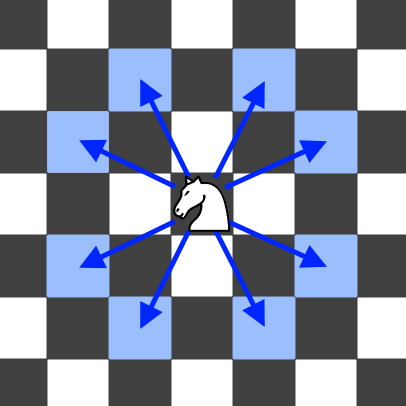688. Knight Probability in Chessboard

On an `N`x`N` chessboard, a knight starts at the `r`-th row and `c`-th column and attempts to make exactly `K` moves. The rows and columns are 0 indexed, so the top-left square is `(0, 0)`, and the bottom-right square is `(N-1, N-1)`.

A chess knight has 8 possible moves it can make, as illustrated below. Each move is two squares in a cardinal direction, then one square in an orthogonal direction.Each time the knight is to move, it chooses one of eight possible moves uniformly at random (even if the piece would go off the chessboard) and moves there.

The knight continues moving until it has made exactly `K` moves or has moved off the chessboard. Return the probability that the knight remains on the board after it has stopped moving.

Example:

```Input: 3, 2, 0, 0
Output: 0.0625
Explanation: There are two moves (to (1,2), (2,1)) that will keep the knight on the board.
From each of those positions, there are also two moves that will keep the knight on the board.
The total probability the knight stays on the board is 0.0625.
```

Note:

• `N` will be between 1 and 25.
• `K` will be between 0 and 100.
• The knight always initially starts on the board.

688. Knight Probability in Chessboard
``````struct Solution;

type Point = (i32, i32);

#[derive(Debug)]
struct Chessboard {
board: Vec<Vec<f64>>,
temp: Vec<Vec<f64>>,
n: i32,
}

impl Chessboard {
fn new(n: i32, p: Point) -> Self {
let m = n as usize;
let mut board: Vec<Vec<f64>> = vec![vec![0.0; m]; m];
let temp: Vec<Vec<f64>> = vec![vec![0.0; m]; m];
board[p.0 as usize][p.1 as usize] = 1.0;
Chessboard { board, temp, n }
}
fn adj(&self, p: Point) -> Vec<Point> {
let offsets = [
(1, 2),
(2, 1),
(2, -1),
(1, -2),
(-1, -2),
(-2, -1),
(-2, 1),
(-1, 2),
];
let mut res: Vec<Point> = vec![];
for offset in &offsets {
let i = p.0 + offset.0;
let j = p.1 + offset.1;
if i < 0 || i >= self.n || j < 0 || j >= self.n {
continue;
}
res.push((i, j));
}
res
}
fn next(&mut self) {
let m = self.n as usize;
for i in 0..m {
for j in 0..m {
let f = self.board[i][j];
if f == 0.0 {
continue;
}
for p in self.adj((i as i32, j as i32)) {
self.temp[p.0 as usize][p.1 as usize] += f;
}
}
}
for i in 0..m {
for j in 0..m {
self.board[i][j] = self.temp[i][j] * 0.125;
self.temp[i][j] = 0.0;
}
}
}
fn sum(&mut self) -> f64 {
let mut res = 0.0;
let m = self.n as usize;
for i in 0..m {
for j in 0..m {
res += self.board[i][j];
}
}
res
}
}

impl Solution {
fn knight_probability(n: i32, k: i32, r: i32, c: i32) -> f64 {
let mut chessboard: Chessboard = Chessboard::new(n, (r, c));
for _ in 0..k {
chessboard.next();
}
chessboard.sum()
}
}

#[test]
fn test() {
use assert_approx_eq::assert_approx_eq;
let n = 3;
let k = 2;
let r = 0;
let c = 0;
let res = 0.0625;
assert_approx_eq!(Solution::knight_probability(n, k, r, c), res);
let n = 3;
let k = 1;
let r = 1;
let c = 1;
let res = 0.0;
assert_approx_eq!(Solution::knight_probability(n, k, r, c), res);
}
``````